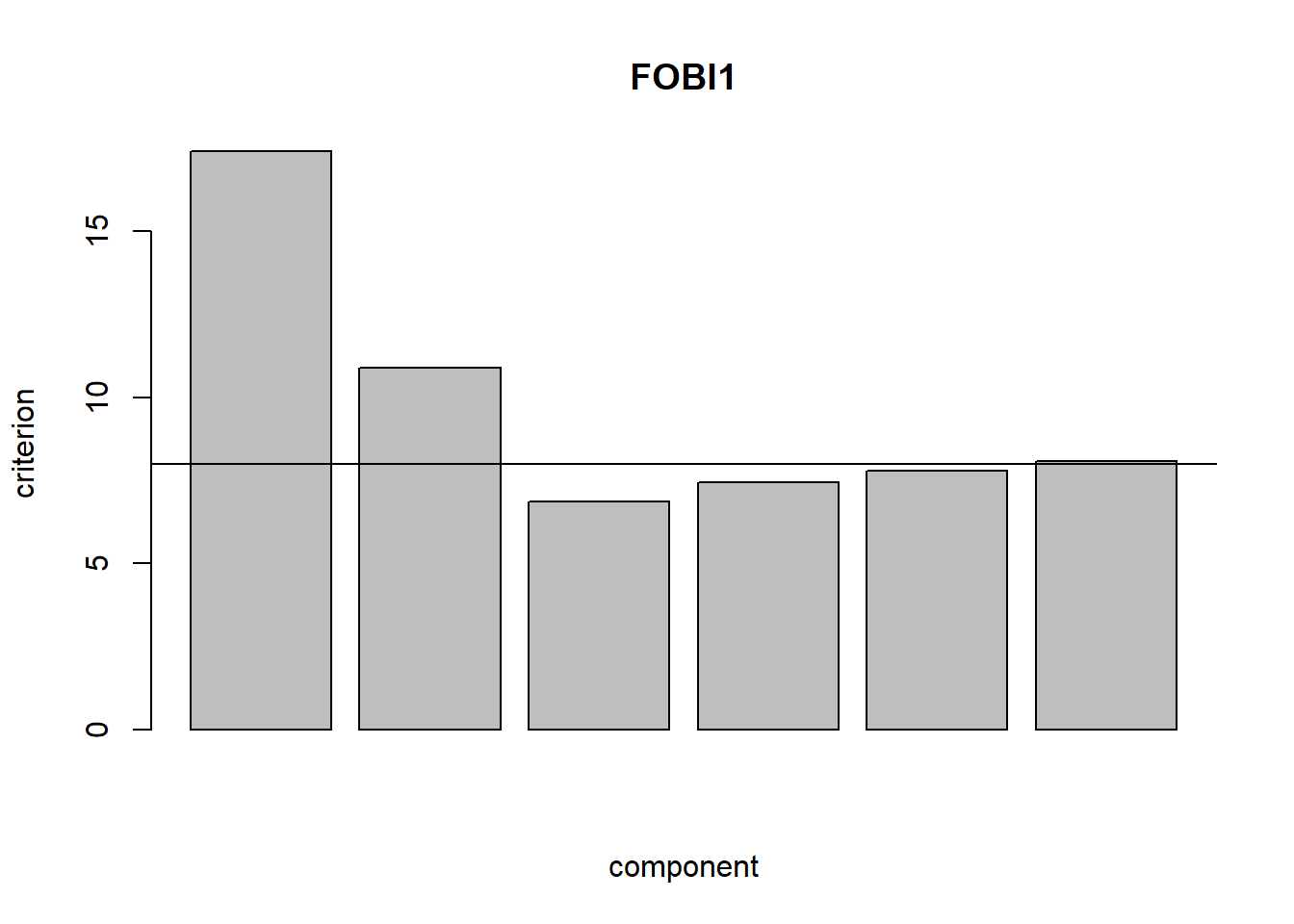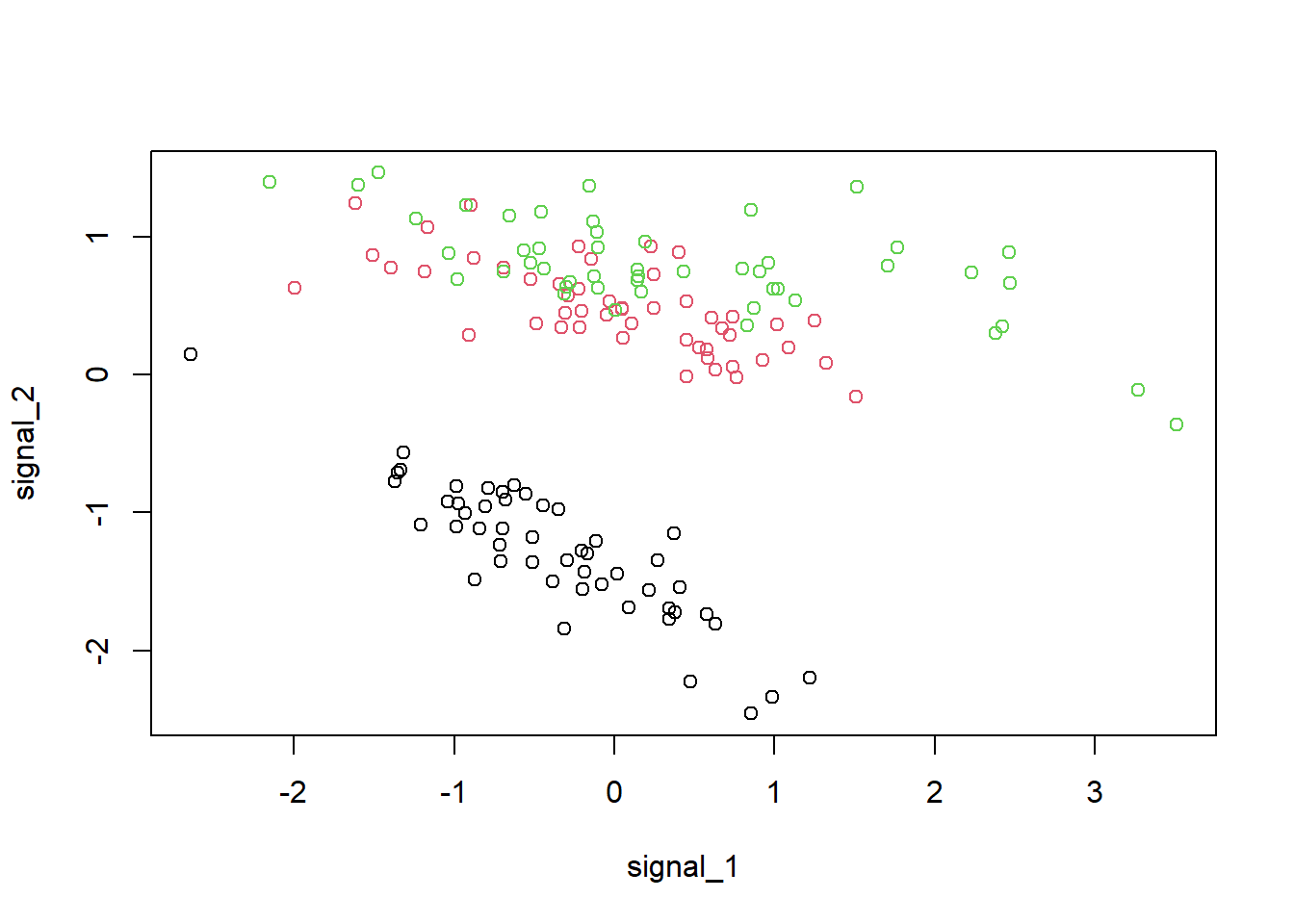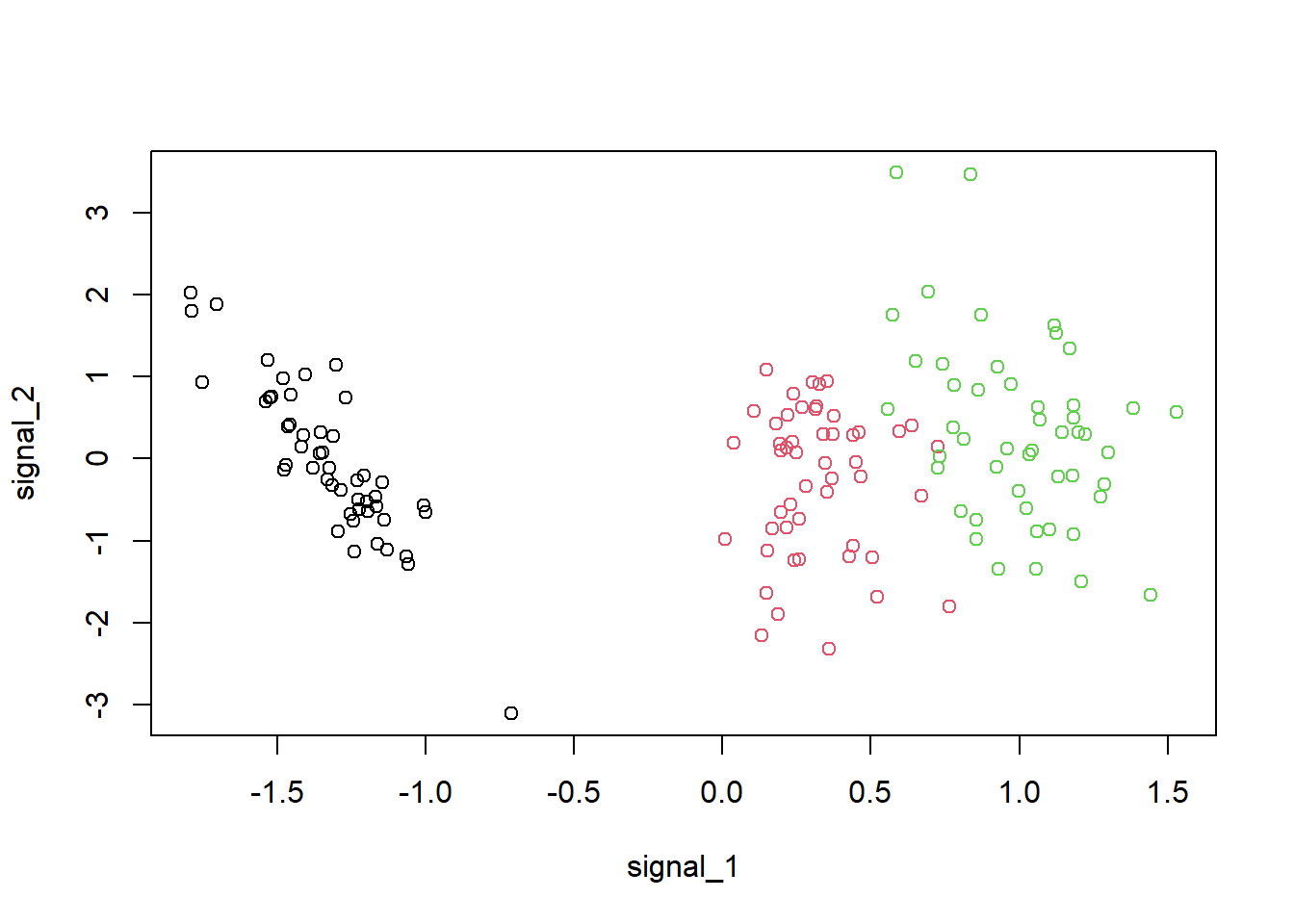## Fourth order blind identification

In independent component analysis (ICA) it is usually assumed that the observable $$p$$-vector $$x$$ is a linear mixture of $$p$$ mutually independent components, where the vector of independent components is $$s$$. Hence the model can be written as $x = As + \mu,$ where the full rank $$A$$ represents the linear mixing and $$\mu$$ the location. It is then usually assumed that $$E(s)=0$$ and $$Cov(s)=I_p$$. The goal is then to find an unmixing matrix $$W$$ such that $$Wx$$ has independent components.

In ICA gaussian components are considered the uninteresting ones whereas the non-gaussian ones are considered interesting. Actually, if there is more than one gaussian component only the non-gaussian components can be recovered but not the gaussian ones.

Therefore it is naturally of interest to test how many interesting components there are. This is done by testing if a subvector of $$Wx$$ is gaussian.

FOBI is one of the first ICA methods - it jointly diagonalizes the regular covariance matrix $$Cov$$ and the so-called matrix of fourth moments $Cov_4(x) = E(r^2(x-\mu)(x-\mu)^T),$ where $$\mu = E(x)$$ and $$r^2=(x-\mu)^T Cov(x)^{-1} (x-\mu)$$.

Note that under this definition $$Cov_4$$ is not consistent under the normal model and the scale of a normal component would be $$\sqrt{p+2}$$. (Therefore for example the function cov4 from the ICS package divides $$Cov_4$$ by $$p+2$$.)

Hence FOBI is the solution to the generalized eigenvector-eigenvalue problem which solves $W Cov(x) W^T = I_p \ and \ W Cov_4(x) W^T = D,$ where D is a diagonal matrix.

For the context under consideration it is natural to order the general eigenvectors in $$W$$ such that the eigenvalues, which are the diagonal elements of $$D$$, $$d_1,...,d_p$$, fulfill $(d_1 - (p+2))^2 \geq ... \geq (d_p - (p+2))^2,$ which means gaussian components would be put at the end of $$s$$ and values of $$d_i$$’s which correspond to a gaussian component should be $$p+2$$.

Hence, assuming that there are $$k$$ interesting components the hypothesis is that $H_0: d_{k+1} = ... = d_p = p+2.$ The package ICtest provides different asymptotic and bootstrapping based tests to test this hypothesis

### Asymptotic tests for a specific value of $$k$$

The function for the asymptotic test is FOBIasymp and it provides three different tests, denoted by S1, S2 and S3, and specified using the type argument:

1. type="S1": The test statistic $$T$$ is the variance of the last $$p-k$$ eigenvalues around p+2: $T = n \sum_{i=k+1}^p (d_i-(p+2))^2$

the limiting distribution of $$T$$ under the null is the sum of two weighted chisquare distributions with weights $$w_1 = 2 \sigma_1 / (p-k)$$ and $$w_2 = 2 \sigma_1 / (p-k) + \sigma_2$$ and degrees of freedom $$df_1 = (p-k-1)(p-k+2)/2$$ and $$df_2 = 1$$.

1. type="S2": Another possible version for the test statistic is a scaled sum of the variance of the eigenvalues around the mean plus the variance around the expected value under normality ($$p+2$$). Denote $$VAR_{dpk}$$ as the variance of the last $$p-k$$ eigenvalues and $$VAR2_{dpk}$$ as the variance of these eigenvalues around $$p+2$$.

Then the test statistic is: $T = (n (p-k) VAR_{dpk}) / (2 \sigma_1) + (n VAR2_{dpk}) / (2 \sigma_1 / (p-k) + \sigma_2)$ This test statistic has a limiting chisquare distribution with $$(p-k-1)(p-q+2)/2 + 1$$ degrees of freedom.

1. type="S3": The third possible test statistic just checks the equality of the last $$p-k$$ eigenvalues using only the first part of the test statistic of type="S2".

The test statistic is then: $T = (n (p-k) VAR_{dpk}) / (2 \sigma_1)$ and has a limiting chisquare distribution with $$(p-k-1)(p-q+2)/2$$ degrees of freedom.

The constants $$\sigma_1$$ and $$\sigma_2$$ depend on the kurtosis values of the independent components and on the dimension $$p$$ and are estimated from the data.

To demonstrate the function FOBIasymp consider the following artificial data

library(ICtest)
set.seed(1)
n <- 1500
S <- cbind(runif(n), rchisq(n, 2), rexp(n), rnorm(n), rnorm(n), rnorm(n))
A <- matrix(rnorm(36), ncol = 6)
X <- S  %*% t(A)

Therefore there are three interesting components and three noise components.

FOBI1 <- FOBIasymp(X, k = 3, model="ICA")
screeplot(FOBI1)
abline(h = 8) # p=6, i.e. p + 2 = 8The screeplot shows that the components are in the right order and 3 components have an eigenvalue close to the value of interest.

The test decision is

FOBI1 
##
##  FOBI subgaussianty test using a chisquare test (Variant II)
##  in an ICA model
##
## data:  X
## T = 4.5359, df = 5, p-value = 0.4751
## alternative hypothesis: there are fewer than 3 gaussian components

Which we can compare to the other test version:

FOBIasymp(X, k = 3, type = "S1", model = "ICA")
##
##  FOBI subgaussianty test using a weighted sum of chisquare test
##  in an ICA model
##
## data:  X
## T = 175.81, w1 = 21.324, df1 = 5.000, w2 = 25.324, df2 = 1.000, p-value
## = 0.2387
## alternative hypothesis: there are fewer than 3 gaussian components
FOBIasymp(X, k = 3, type = "S2", model = "ICA")
##
##  FOBI subgaussianty test using a chisquare test (Variant I)
##  in an ICA model
##
## data:  X
## T = 7.6589, df = 6, p-value = 0.2642
## alternative hypothesis: there are fewer than 3 gaussian components

which means all three tests do not reject that the last three components are gaussian. Using the default, model = "NGCA" should be also consistent but does not explicitely assume an ICA model but the more general non-gaussian component analysis (NGCA) model with possible dependent interesting signals.

To see if there are 4 gaussian components using the default model argument one could use for example:

FOBIasymp(X, k = 2)
##
##  FOBI subgaussianty test using a chisquare test (Variant II)
##  in an NGCA model
##
## data:  X
## T = 20.455, df = 9, p-value = 0.01531
## alternative hypothesis: there are fewer than 4 gaussian components

which would be rejected at the $$0.05$$ level.

### Bootstrapping tests for a specific value of $$k$$

The test statistic used here is again the variance of the last $$p-k$$ components around the value of interest $$p+2$$: $T = n \sum_{i=k+1}^p (d_i-(p+2))^2$ Two possible bootstrap testing strategies, denoted B1 and B2, are provided for testing the null hypothesis of interest.

Let $$X$$ be the centered data matrix of the $$n$$ $$p$$-variate observations and $$S$$ the corresponding estimated independent components which can be decomposed into $$S_1$$, the non-gaussian components and $$S_2$$ the gaussian components. Let $$W$$ be the estimated unmixing matrix.

1. s.boot="B1": The first strategy has the following steps:
1. Take a bootstrap sample $$S_1^*$$ of size $$n$$ from $$S_1$$.
2. Take a bootstrap sample $$S_2^*$$ consisting of a matrix of standard normally distributed elements.
3. Combine $$S^*=(S_1^*, S_2^*)$$ and create $$X^*= S^* W^{-1}$$.
4. Compute the test statistic $$T^*$$ based on $$X^*$$.
5. Repeat the previous steps n.boot times to get the test statistics $$T^*_1,..., T^*_{n.boot}$$.

Note that in this bootstrapping test the assumption of ‘’independent components’’ is not used, it is only used that the last $$p-k$$ components are gaussian and independent from the first $$k$$ components. Therefore this strategy can be applied in an independent component analysis (ICA) framework and in a non-gaussian components analysis (NGCA) framework.

1. s.boot="B2": The second strategy has the following steps:
1. Take a bootstrap sample $$S_1^*$$ of size $$n$$ from $$S_1$$ where the subsampling is done separately for each independent component.
2. Take a bootstrap sample $$S_2^*$$ consisting of a matrix of standard normally distributed elements.
3. Combine $$S^*=(S_1^*, S_2^*)$$ and create $$X^*= S^* W^{-1}$$.
4. Compute the test statistic $$T^*$$ based on $$X^*$$.
5. Repeat the previous steps n.boot times to get the test statistics $$T^*_1,..., T^*_{n.boot}$$.

The p-value is then in both cases $\frac{\#(T_i^* \geq T)+1}{n.boot+1}.$

This bootstrapping strategy assumes a full ICA model and cannot be used in an NGCA framework.

To test for the previous data whether there are three gaussian components, the bootstrap FOBIboot can be used as follows:

FOBIboot(X, k = 3, s.boot = "B1")
##
##  ICA subgaussianity bootstrapping test using FOBI and strategy B1
##
## data:  X
## T = 527.43, replications = 200, p-value = 0.194
## alternative hypothesis: the last 3 components are not gaussian
FOBIboot(X, k = 3, s.boot = "B2")
##
##  ICA subgaussianity bootstrapping test using FOBI and strategy B2
##
## data:  X
## T = 527.43, replications = 200, p-value = 0.1493
## alternative hypothesis: the last 3 components are not gaussian

## Non-Gaussian projection pursuit

Non-Gaussian projection pursuit (NGPP) assumes that the observed multivariate data is generated by a linear mixture of $$k$$ signals and $$p - k$$ channels of Gaussian noise. The package provides both methods for estimating the signals when $$k$$ is known a priori and a technique for testing and estimating the value of $$k$$ itself. The estimation is based on the maximization of convex combinations of squared objective functions, of which four popular ones are included in the package:

• Third cumulant, skew
• Fourth cumulant, pow3
• Logarithmic hyperbolic cosine, tanh
• Gaussian function, gauss

The names (skew etc.) of the functions in the package are based on the corresponding derivatives, or non-linearities.

### Estimating the signal components

The simplest way to use NGPP is to just call the function using default settings and indicating the number of signal components k you want to extract. The default objective function is a certain linear combination of squared third and fourth cumulants, the Jarque-Bera test statistic for normality. To use some other objective function you can specify a vector of objective function names in nl and the corresponding weights in alpha.

library(ICtest)
X <- as.matrix(iris[, 1:4])

res1 <- NGPP(X, k = 2)
plot(res1$S, col = as.factor(iris[, 5]), xlab = "signal_1", ylab = "signal_2") res2 <- NGPP(X, k = 2, nl=c("tanh", "pow3"), alpha = c(0.5, 0.5)) plot(res2$S, col = as.factor(iris[, 5]), xlab = "signal_1", ylab = "signal_2")### Testing for a specific value of $$k$$

The above example used the default method="symm" to specify that all k signals should be estimated simultaneously and the alternative method="defl" could have been used to estimate the signals one-by-one, leading into different solutions. But perhaps more interestingly, the one-by-one estimation can also be used to test the null hypothesis $$H_0: true\_k \leq k$$ with the function NGPPsim. Let’s create next some mixed toy data and try to infer its true signal dimension.

set.seed(2016)
S <- cbind(rexp(100), rnorm(100), rnorm(100))
A <- matrix(rnorm(9), 3, 3)
X <- S%*%A
res0 <- NGPPsim(X, k = 0, N = 200)
res0$p.value ##  0 res1 <- NGPPsim(X, k = 1, N = 200) res1$p.value
##  0.935

The obtained $$p$$-values provide sufficient evidence to conclude that $$k\_true > 0$$ and $$k\_true \leq 1$$, correctly implying that the signal dimension is equal to one. The parameter N controls the number of repetitions used to simulate the distribution of the test statistic under the null hypothesis and increasing it gives more accurate results but increases the computation time.

### Estimation of the true dimension

In the above example we had to separately call NGPPsim for each value of k. This process is automated by the function NGPPest which performs the hypothesis test for all $$k = 0, \ldots , p-1$$. Let’s create another toy data and try to estimate its dimension, using this time only the non-linearity pow3.

set.seed(2016)
S <- cbind(rexp(100), runif(100), rnorm(100))
A <- matrix(rnorm(9), 3, 3)
X <- S%*%A
res <- NGPPest(X, nl = "pow3", N = 200)
res\$p.value
##  0.000 0.080 0.855

The resulting vector of $$p$$-values can now be directly interpreted as testing the null hypotheses whether each particular component is just noise. The correct conclusion of two signal components is again reached.